# Pricing management : applying discounts (Simple discount, Quantity discount)Posted by

Let’s continue exploring the Pricing Management module through Discounts. Before going in further details, please have a look on the previous post relating this feature :

Discounts encompass a lot of price capabilities such as simple discount, quantity discount, Threshold discount, free item, mix and match discount and coupon. That is much more than the previous sales trade agreement combined with trade allowance management.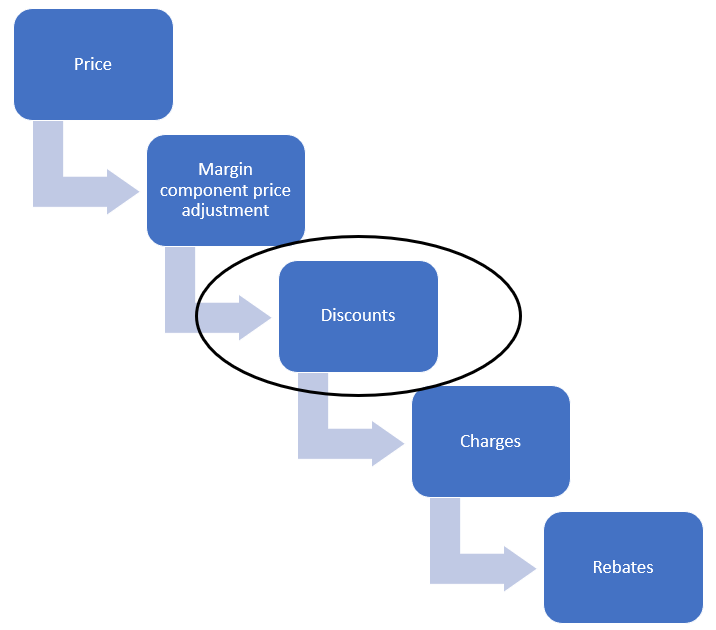Discounts are applied just after the Base price potentially updated with a margin adjustment. The way discounts are combined and apply between them will be addressed here with the default discount concurrency mode.

# Data sample

The data set used is the following :

• 2 customers with 2 different customer groups
• 2 items :
• Bike 1 (base price 150 \$ adjusted of +10 \$) with following attribute value : Application = mix; Option = medium
• Bike 2 (base price 150 \$ adjusted of +20 \$) with following attribute value : Application = mountain; Option = medium

From a sales order, without any discount so far, the result is :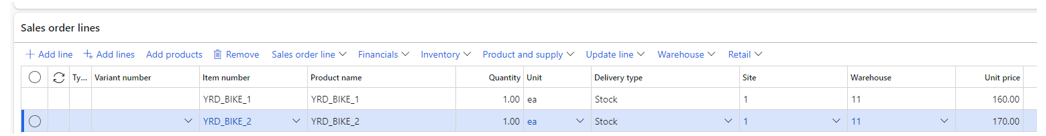Every discount is setup the same way : you will need to register a discount from Pricing management > During-sales pricing > Discounts, choose the appropriate discount, setup it properly and enable it.

# Simple Discount

Let’s start by this one. With this discount type you can apply a discount amount or percentage from the Adjusted base price.

In this scenario I want to apply a percentage discount on each bike.

## Use case 1 – Compounded

Before creating the discount, you need to have a Discount price component, so let’s go for creating a new Price component code. As you can see, the Price component is setup to Discounts and the Default discount concurrency mode is setup to Compounded.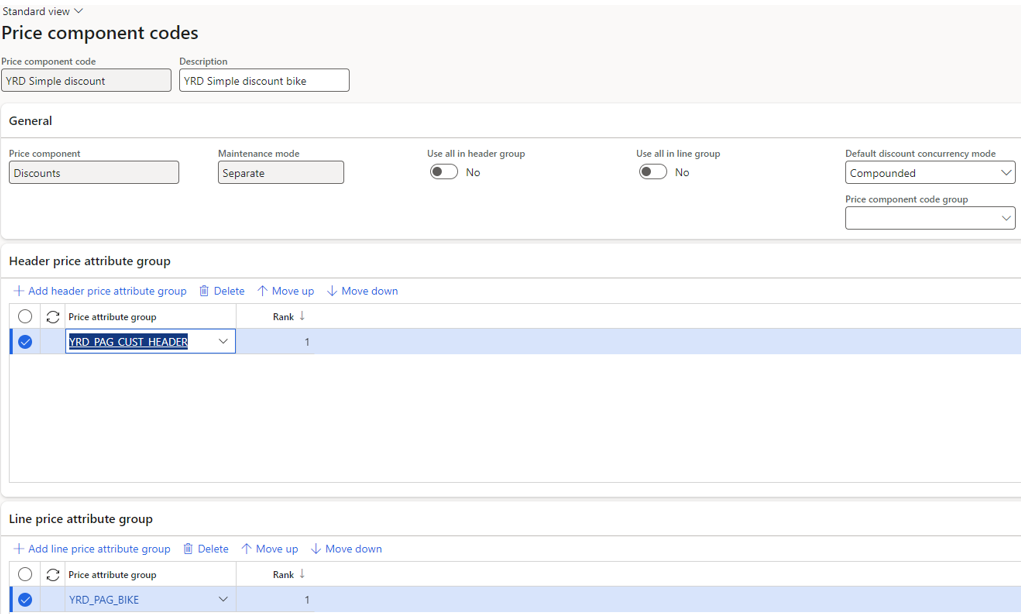I have retrieved the price attribute groups setup previously, for making eligible my customers and my bike items.

Then, you will need to create the discount itself.

Go under Pricing Management > During-sales pricing > Discounts > Discounts and create a new discount.

You can notice the Discount concurrency mode is also compounded, and the Price component code setup is the one just created. Note that the policy setup in the discount override the one on the Price component code.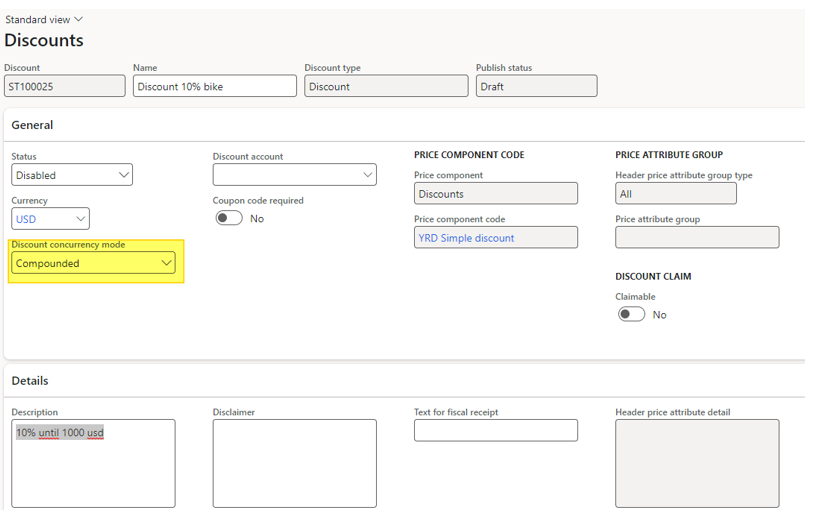On the header price attribute group, I’ve made a filter on the 2 customers groups.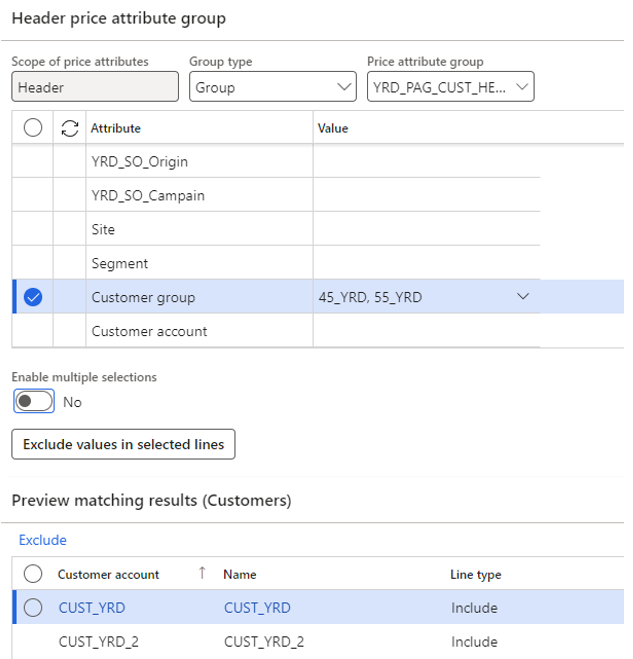If the customer filter doesn’t matter to you, you should select the Use all in header group. Under Price/discount tab, I’ve setup the Percentage of 5%. You can also use the quantity limit field if needed. Don’t forget the Validation period.

Then, you can add lines and edit the line price attribute.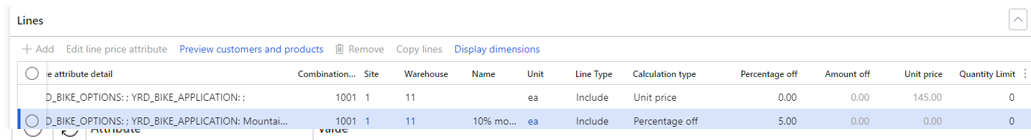Here I’ve put a unit price of  145 \$ (both bikes) and 5% off for bike2.

After enabling the discount, you have to complete the trees for discount with the appropriate price component code and pricing sequence.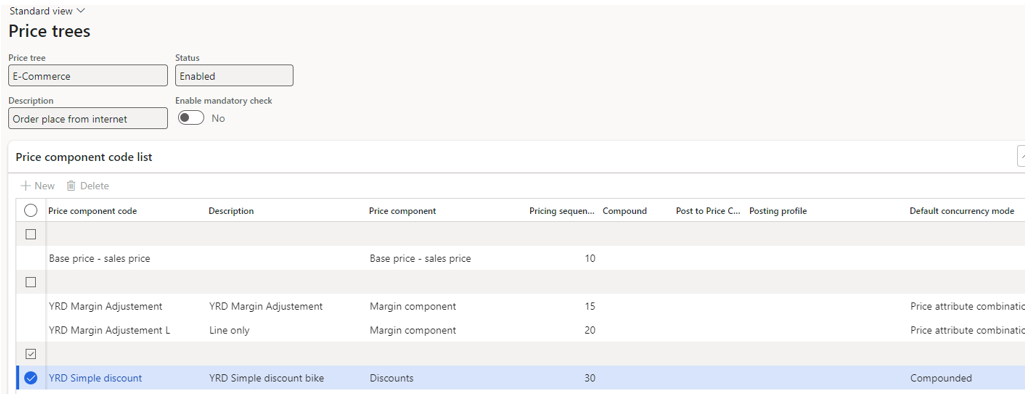What’s the result ?  By creating a sales order, price calculated are setup to 145 \$ for each bike.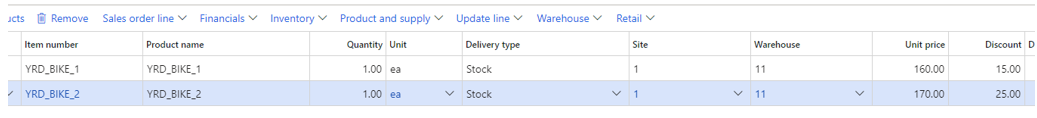The best price is applied within the same discount.

## Use case 2 – Compounded with 2 discounts

Now let’s test by applying 2 different discounts.

For the first one I have 5% off for bike2 and 10% off on both bikes with the second line.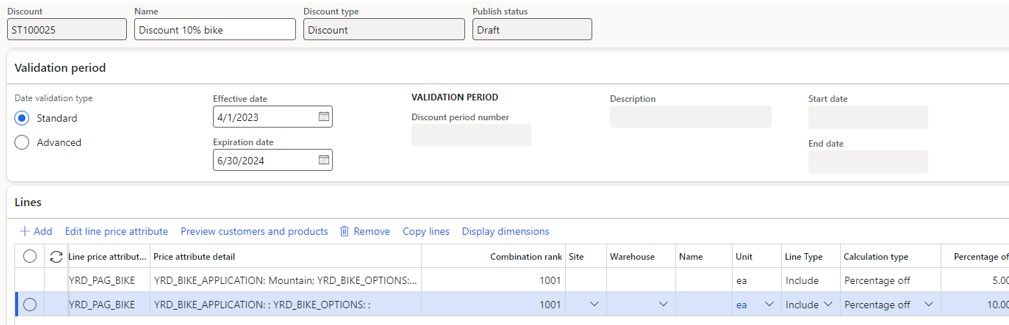Creating a new discount, with the same criteria, but different percentage off : 20% for bike 2 and 15% on both bikes.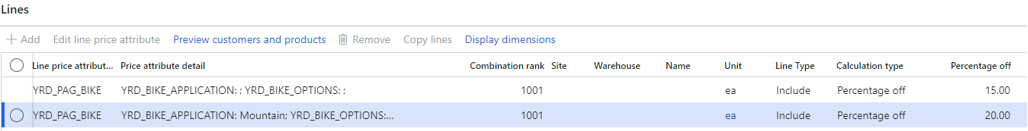What are the results ? On the sales order line, the price are the following: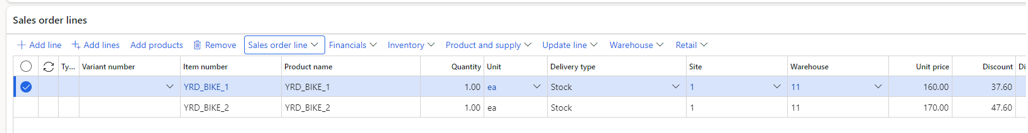Bike1 : 10% discount + 15% discount = 160 x -10% x -15% = 122.4 = 160 – 37.60

The 2 discount are applied in a compounded way (cascade). See price detail :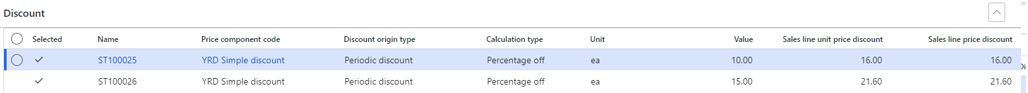Bike2 : 10% discount + 20% discount = 170 x -10% x -20% = 122.4 = 170 – 47.60

Same result. For the first discount, the engine applied the best eligible discount (10% > 5%) and then compounded with the second discount with the best eligible discount (20% > 15%).

## Use case 3 – Best price

Now let’s consider using the best price for discount concurrency mode. I’m updating the two discounts by changing this value

The result is the following, for bike2 :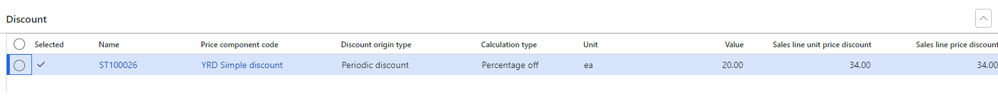The best discount is applied, meaning here the ST100026 because 20% is the best discount comparing to the 10% setup in the other applicable discount.

## Use case 3 – Exclusive

By adding a new Exclusive discount for bike2, making a total of 3 eligible discounts now, let’s see what do we have.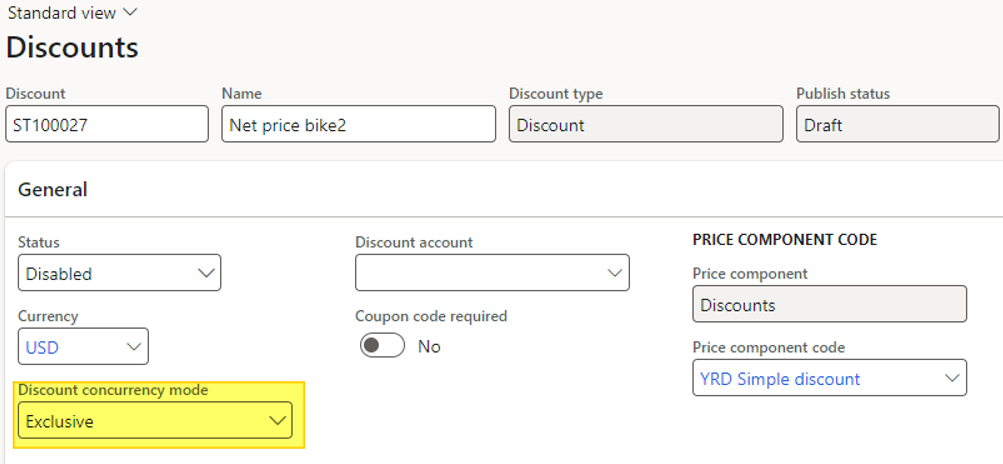I have setup a unit price exclusive of 120 \$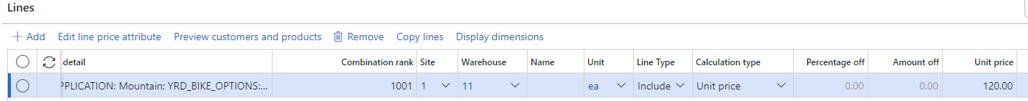The result is the following on a sales order line :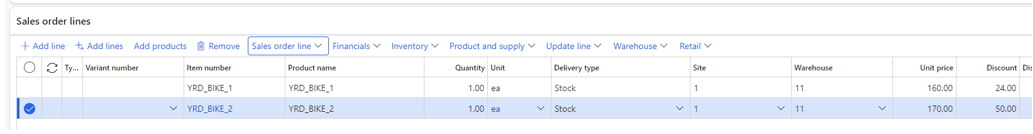The price is calculated to 120 \$ (170 – 50). Having a look at the price detail :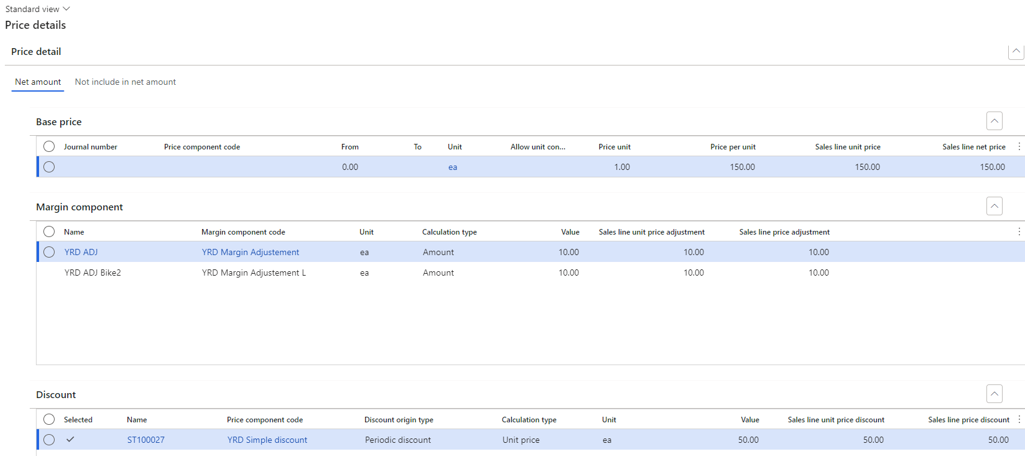Only one discount is retrieved. It’s the best way to setup net prices when it’s required.

### Use case 4 – Always apply

Another discount concurrency mode is Always apply. Let’s create a new discount with this discount concurrency mode and an amount off of 1 \$.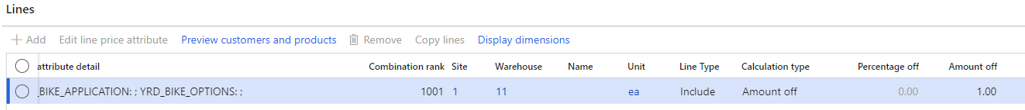The Always apply…always applied. Even if there is some exclusive discount, any always apply discount will be retrieved. Pay attention Always apply concurrency mode does not apply on every discount type (mix-and-match for instance).

Let’s see the results on the sales order line :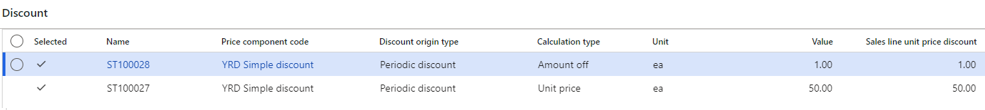Even if there is an exclusive price, the 1\$ additional (always apply) discount is applied at the end of calculation.

## Quantity discount

The quantity discount is the second type of discount available. You can setup an amount or a percentage discount from the price with requirement of a certain quantity purchased by the customer to trigger the discount.

Let’s disable the Exclusive discount, and put the two previous simple discount in a compounded mode.

Now, let’s add a quantity discount with the same header / line price attribute groups.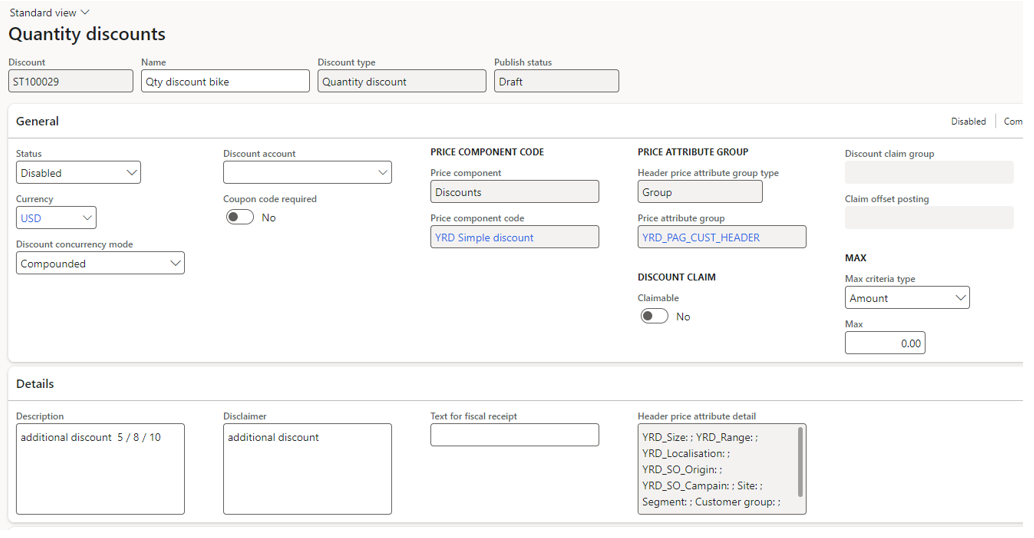### Use case 1 – Percentage off

Under the Quantity discount configuration, let’s configure the calculation type with Percentage off.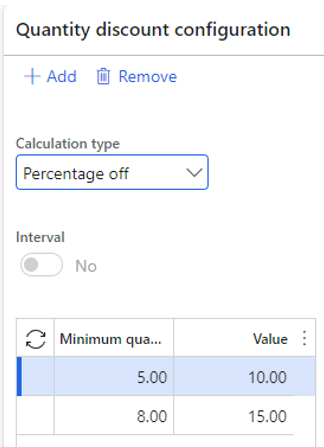You can notice the 2 minimum quantity setup : by purchasing 5 units, the percentage applied is 10%, and up to 10% from 8 units purchased.

By creating a new sales order for 1 bike…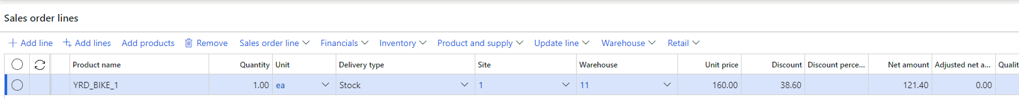… the calculated price is the following for bike1 : 160 – 38.6 = 121.4 \$ = 160 – 10% (simple discount) x -15% (simple discount)  – 1 (always apply discount)

But changing the quantity to 5 units :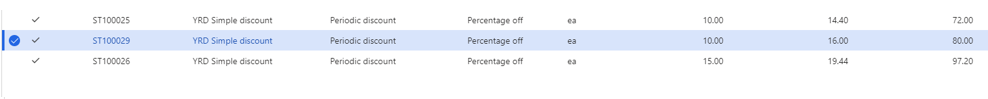bike1 : 160 – 38.6 = 160 – 10% (simple discount) x -15% (simple discount)  x -10% (quantity discount) – 1 (always apply discount) = 109.16 \$

The quantity discount is triggered as expected. The triggers apply also when the sum of quantity for the item on lines reach the minimum.

Note : if the 2 simple discounts are setup in Best price approach, the Quantity discount in the compounded approach will not be triggered. All discounts have to be in the same approach for concurrency (except Always apply, Exclusive).

### Use case 2 – Amount off

Testing now the Amount off approach on the quantity discount :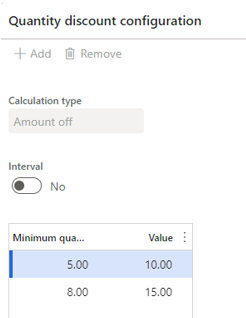The result on sales order line, with a quantity of 5 units, is the following :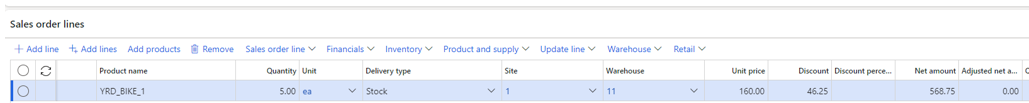The unit price applied is 113.75 \$. Having a look at the price detail :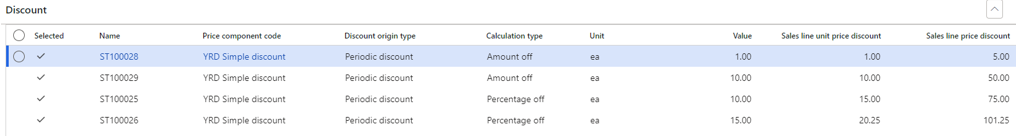Bike1 : 160\$ – 10\$ (quantity discount) = 150 \$ – 10% (simple discount) x -15% (simple discount)  – 1 (always apply discount) = 113.75 \$

By changing the quantity up to 10 units, look at the results: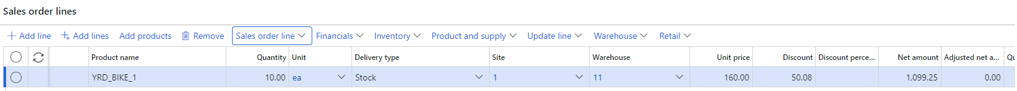Bike1 : 160\$ – 15\$ (quantity discount) = 145 \$ – 10% (simple discount) x -15% (simple discount)  – 1 (always apply discount) = 109.82 \$

You have notice that Amount off is applied first (except the Always apply, at the end).

### Use case 3 – Interval

Let’s activate the interval checkbox to see the difference.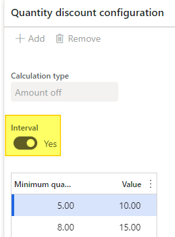With a sales order line for a quantity of 6, looking at the price details :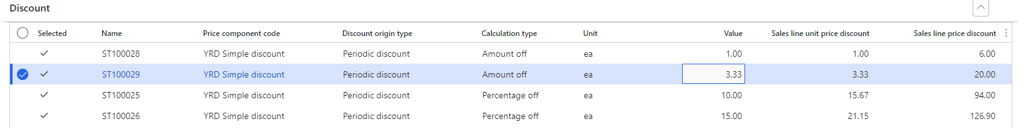The quantity discount applied is 20 (6 units x 3.33).

The explanation of interval is the following :

• First item to 4th item ordered: no quantity discount
• 5th item ordered to the 7th : 10 \$ each

In my case, 2 are eligible for a discount, making a total of 20 \$

By filling a quantity of 10 units, look at the results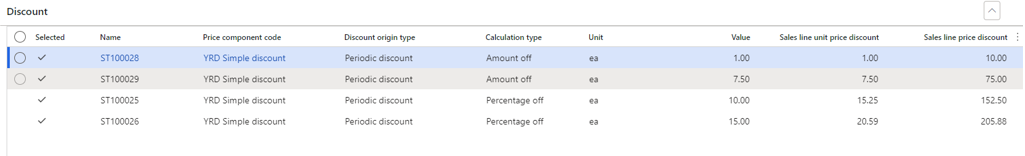• First item to 4th item ordered: no quantity discount
• 5th item ordered to the 7th : 10 \$ each making a total of 30 \$
• 8th item ordered to the 10th : 15 \$ each making a total of 45 \$

Finally, the discount is 75 \$ (30 + 45), so 7.50 per line.

### Use case 4 – several lines

Let’s disable interval and have some attention to the trigger on several lines. Remember the trigger is setup from 5 units.

On the first sales order line, I’ve put a quantity of 2 : no quantity discount is applied.

On the second line, the quantity is 4 : the sum of items has reached the trigger. We can notice the discount is higher on the second line.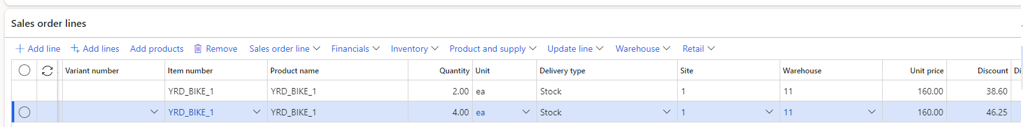Any update (clic save, or sales order line, or go back to the first line, or clic recalculate…) on the sales order will impact the first line.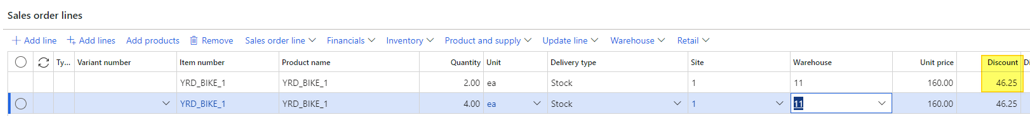That’s cool because this will have importance for mix-and-match discount too.

That’s it for today.

Yohann